# 题目地址

http://poj.org/problem?id=1191

# 题目大意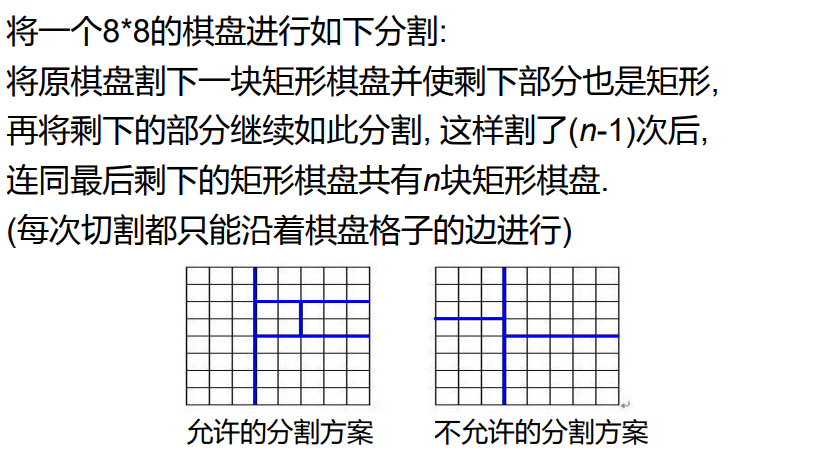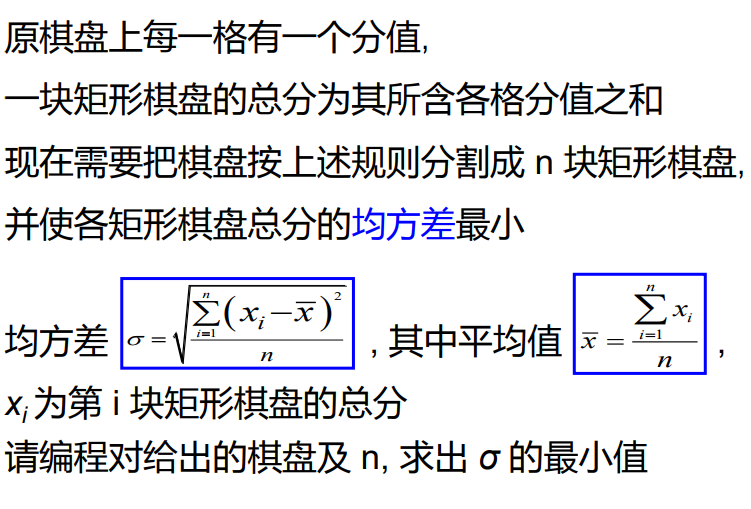# 解题思路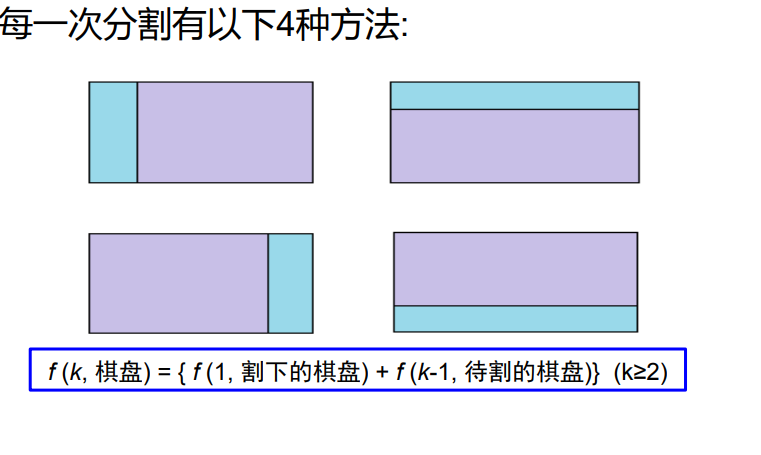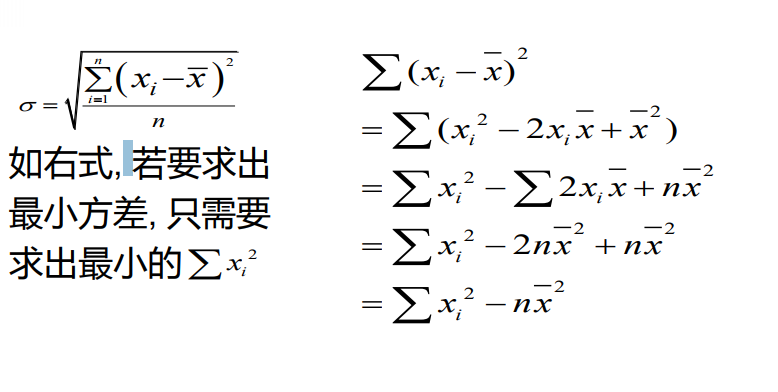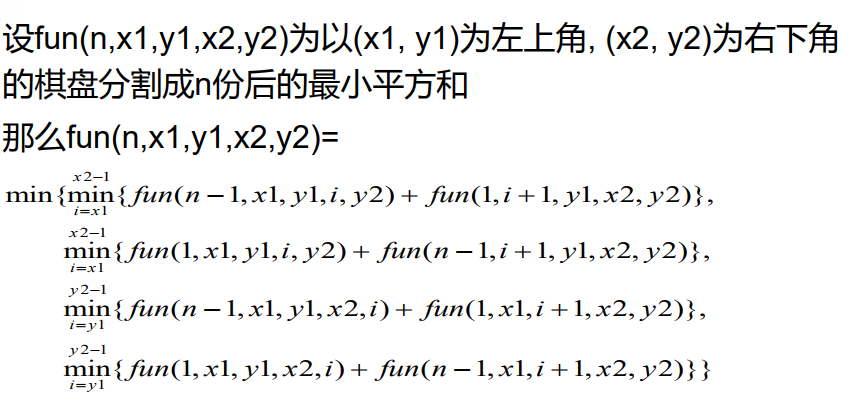# Code

``````
#include <stdio.h>
#include <iostream>
#include <stdlib.h>
#include <string.h>
#include <queue>
#include <map>
#include <vector>
#include <math.h>
#include <algorithm>
#define INF 0x3fffffff
#define N 80

using namespace std;
typedef long long LL;

int n;
int s; //每个格子的分数
int sum; //(1,1)到(i,j)的矩形的分数之和
int res; //fun的记录表

//(x1,y1)到(x2,y2)的矩形的分数之和
int get_sum(int x1, int y1, int x2, int y2) {
return sum[x2][y2] - sum[x2][y1-1] - sum[x1-1][y2] + sum[x1-1][y1-1];
}

int fun(int n, int x1, int y1, int x2, int y2) {
if (res[n][x1][y1][x2][y2] != -1) {
return res[n][x1][y1][x2][y2];
}
if (n == 1) {
int ans = get_sum(x1, y1, x2, y2);
res[n][x1][y1][x2][y2] = ans*ans;
return res[n][x1][y1][x2][y2];
}
int MIN = INF;
for (int x = x1; x < x2; x++) {
int up = get_sum(x1, y1, x, y2);
int down = get_sum(x+1, y1, x2, y2);
int local = min(fun(n-1, x1, y1, x, y2) + down*down, fun(n-1, x+1, y1, x2, y2) + up*up);
MIN = min(MIN, local);
}
for (int y = y1; y < y2; y++) {
int left = get_sum(x1, y1, x2, y);
int right = get_sum(x1, y+1, x2, y2);
int local = min(fun(n-1, x1, y1, x2, y) + right*right, fun(n-1, x1, y+1, x2, y2) + left*left);
MIN = min(MIN, local);
}
res[n][x1][y1][x2][y2] = MIN;
return MIN;
}

int main() {

#ifndef ONLINE_JUDGE
freopen("in.txt", "r", stdin);
#else
//
#endif
scanf("%d", &n);
memset(sum, 0, sizeof(sum));
memset(res, -1, sizeof(res));
for (int i = 1; i < 9; i++) {
int row_sum = 0;
for (int j = 1; j < 9; j++) {
scanf("%d", &s[i][j]);
row_sum += s[i][j];
sum[i][j] = sum[i-1][j] + row_sum;
}
}
double ans = fun(n, 1, 1, 8, 8) - 1.0 * (sum*sum) / n;
ans = sqrt(ans/n);
printf("%.3f\n", ans);
return 0;
}
``````

# 参考

https://d396qusza40orc.cloudfront.net/pkupop/lectures/Week12/W12-03_%E9%80%92%E5%BD%92-%E6%A3%8B%E7%9B%98%E5%88%86%E5%89%B2.pdf

### 虚拟机ubuntu使用串口_weixin_30348519的博客-程序员秘密

1. 电脑的串口默认是在windows系统上，需要把串口转到ubuntu上面，按照下面的步骤先2. 找到需要使用的串口3. 在VMWARE里面连接该串口或者使用方法4. 成功之后，检查一下ls /dev/tty*，最后两个就是映射出来的串口转载于:https://www.cnblogs.com/429512065qhq/p/9401286.html...

### hive 中 json 字符串解析之 get_json_object 与 json_tuple_get_jason_冰阔落的博客-程序员秘密

在技术对app进行埋点时，会讲多个字段存放在一个数组中，因此模型调用数据时，要对埋点数据进行解析，以作进一步的清洗。本文将介绍解析json字符串的两个函数：get_json_object和json_tuple。表结构如下：一、get_json_object函数的作用：用来解析json字符串的一个字段：select get_json_object(flist,'\$.fi...

### 二叉查找树(BST)_(bst-lchild,x)_大脸猫Coding的博客-程序员秘密

title: 二叉查找树(BST)date: 2020-01-13 20:36:30tags: 数据结构1.1二叉查找树定义二叉查找树（Binary Search Tree, BST）是特殊的二叉树，又称排序二叉树、二叉搜索树、二叉排序树。递归定义：①可为一棵空树②非空则由根结点、左子树、右子树组成。左右子树均为一棵二叉查找树，且根结点、根左孩子、根右孩子大小为 左孩子&lt;=根...

### Redis专题详细教程（二）_浩哥要努力的博客-程序员秘密

Redis持久化：RDB触发机制：1.save的规则满足的情况下，会自动触发rdb规则2.执行flushall命令，也会触发rdb规则3.退出redis，也会产生rdb文件备份就会自动生成一个rdb文件dump.rdb恢复rdb文件，只需要将dump.rdb文件放到redis 的启动目录（config get dir命令查看，一般为/usr/local/bin）下就行了，redis启动时会自动检查dump.rdb恢复其中的数据优点：1.适合大规模的数据恢复2.对数据的完整星要求不高